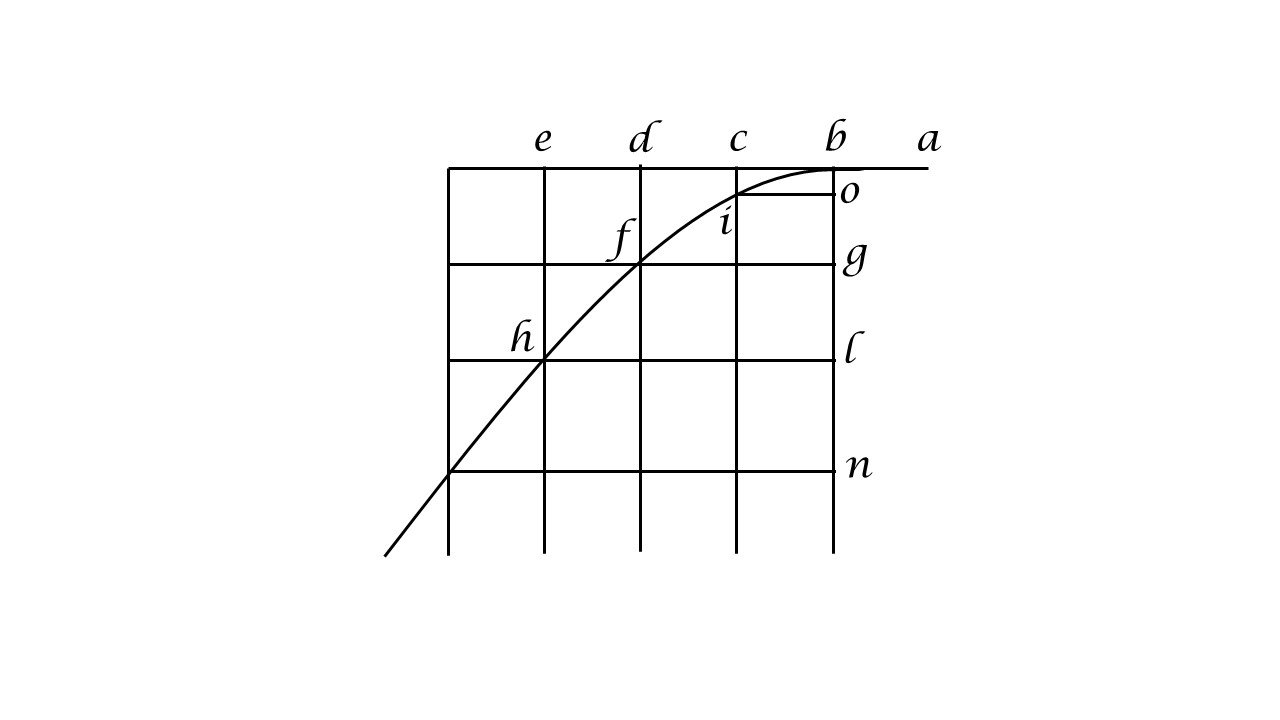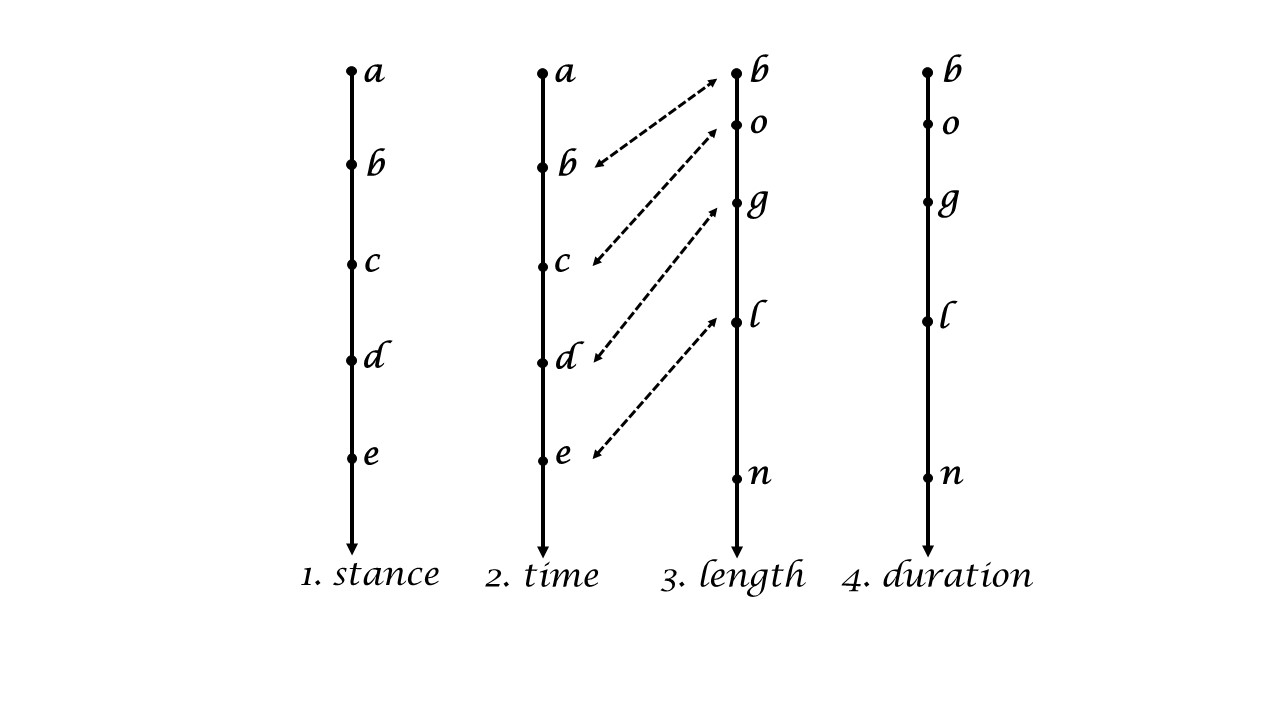iSoul In the beginning is reality.

# Motion ordered by time or stance

This continues posts about Galileo such as here. Look again at this figure from Galileo:The horizontal line represents the independent variable, which is the horizontal component of a projectile with an initial speed that falls with uniform acceleration. The independent variable need not be horizontal, so let us represent it vertically with the vertical component of motion:The first two lines represent the independent uniform motion but are measured differently, (1) by stance and (2) by time. The second and third lines show the correspondence between the independent variable (time) and the dependent variable (length). The first and fourth lines have the same relation: stance is the independent variable and duration is the dependent variable. Lines 1 and 2 are proportional, as are lines 3 and 4. As the units change, the functional relationship does not: the dependent variable is proportional to a square of the independent variable.

Time is the measure of duration of a uniform motion that is the reference motion, which means it is the independent variable. Similarly, stance is the measure of length of a uniform motion that is the reference motion, in which case it is the independent variable. The dependent variable for stance is duration in three dimensions, whereas for time the dependent variable is length in three dimensions.